# Secret Sharing with Reduced Share Size and Data Integrity

Secret Sharing with Reduced Share Size and Data Integrity
Vignan’s Foundation for Science, Technology and Research, Vadlamudi 522213, Guntur Dist., Andhra Pradesh, India

Vignan’s LARA Institute of Technology and Science, Vadlamudi 522213, Guntur Dist., Andhra Pradesh, India

Corresponding Author Email:
prashantiguttikonda77@gmail.com
Page:
227-237
|
DOI:
https://doi.org/10.18280/isi.250210
6 December 2019
|
Accepted:
11 February 2020
|
Published:
15 May 2020
| Citation

OPEN ACCESS

Abstract:

The proposed work is to provide security for audio data stored in cloud data centers and online song repositories such as hungama.com, iTunes, where huge number of songs is stored for listening and downloading. When polynomial secret sharing method is applied on secret audio, audio shares are generated each with the size of secret audio. Secret is derived only when a valid subset of members in the group are compromised. The purpose of the proposed work is to reduce the dimensions of shares. This can be achieved by having the amplitude values of the secret audio as the coefficients of the polynomial instead of random values in polynomial based secret sharing scheme. But having amplitude values as coefficients does not generate meaningless shares and reveals information about the secret. In this paper, two methods are proposed for generating meaningless audio shares such that the dimensions of each share are lesser than the dimensions of confidential audio. It gives dealer an advantage to securely store and distribute the shares by hiding the shares into other media. In the first method, secret audio is encrypted and the values of these encrypted audio are taken as the coefficients of the polynomial to generate the shares. Second method is implemented to reduce the burden of encryption and decryption. In this approach, for share construction the leading term in the polynomial will have random values as coefficient and for all other terms the coefficient are amplitude values of the secret audio. In both methods, shares generated are of reduced dimensions and are meaningless. Security of shares is enhanced by providing integrity mechanism to shares and steganography effect. A novel block cipher is implemented as compression function in the hash algorithm to generate checksum which is used for verifying integrity of shares.

Keywords:

audio shares, integrity mechanism, Lagrange’s interpolation, polynomial, secret sharing, steganography

1. Introduction

As the usage of internet is growing, it is becoming more essential to secure every aspect of online information and data. In this regard, many encryption and watermarking algorithms are developed to tackle several issues related to security. But there are some situations that secret has to be distributed and controlled among a group of participants. That is instead of keeping the secret with one person, portions of the secret are given to the members of the group. To deal with such scenarios Shamir  and Barkely  individually introduced an approach where the confidential data is cleaved into meaningless parts which are allocated to the members of the group. Each part does not reveal any information and are distributed to the participants. Only combination of any few parts reveals the information. Visual cryptography scheme developed by Moni Naor et al.  for binary images require simple computations while retrieving the secret. Based on VC scheme Ateniese et al. established general access structure . Blundo et al.  analyzed the (k,n) VCS in terms of contrast of the reconstructed image and proposed (2,n) scheme for optimal contrast and minimal pixel expansion and Hofmeister et al.  has extended this to (k,n) scheme. Duo Jin et al. designed microblock encoding scheme and halftoning technique which allows visual cryptography on binary, gray scale and color images . Thenin and Lin  proposed a technique where the coefficients of polynomial are the pixel values. This secret sharing scheme reduced the size of shares generated. Lin and Tsai  presented a procedure where after the shares are generated, they are further processed by embedding them into camouflage images and also provide detection of false participants before reconstruction is performed . They also developed a method  where the dimension of confidential data is reduced by converting original image into frequency domain with discrete cosine transform. Wang et al.  proposed (2,n) secret scheme with scalable property which has generalized to (k,n) secret schemes by Yang and Huang . Dong Xie et al.  proposed a scheme that includes additional properties of flexibility, non-resilient along with smooth scalability.

Nowadays audio has also become one of important multimedia content that contain confidential information. Call centers often record their customer interactions that may have sensitive information like credit card numbers, addresses etc. These call centers hire cloud data centers to store their data. An intruder of cloud data center or unauthorized person may use this confidential data for his own benefit. Thus, providing security to such sensitive information has become very important. In such scenarios secret sharing on audio is employed.

Similar to images confidential audios can be encrypted into shares and distributed among cloud data centers. As Simultaneous playing of few shares reveals the secret audio. Unless required number of cloud data centers is compromised, an adversary cannot get the secret. Yvo et al. proposed audio secret sharing scheme which uses sound inference property to embed messages into music and this scheme has been limited to (2, n) threshold . Daniel and Spyros  proposed visual cryptography scheme for audio with the existing general access structure. Huan et al. [17, 18] embedded the n shares generated into n shelter audios thereby improving the security of the ASS scheme. The audio sharing schemes described above generate shares but do not provide any additional features of authentication, integrity and steganography. We proposed methods that not only generate audio shares of reduced dimensions but also provide integrity and steganographic effect to the shares.

Our Proposed method is related to (k,n) threshold method developed by shamir. This method assumes that secret S is divided into shares S(1), S(2), …, S(n). Let k be the minimum shares required to retrieve the secret and n be the number of participants to whom shares has to be distributed. m1, m2, m3, …, mk are random numbers and q be prime number. With secret S as constant term and m1, m2, m3…. mk as coefficients, (k-1) degree polynomial is constructed.

Our Proposed method is related to (k,n) threshold method developed by Shamir. This method assumes that secret S is divided into shares S(1), S(2), …, S(n). Let k be the minimum shares required to retrieve the secret and n be the number of participants to whom shares has to be distributed. m1, m2, m3…. mk are random numbers and q be prime number. With secret S as constant term and m1, m2, m3, …, mk as coefficients, (k-1) degree polynomial is constructed.

$f(x)=\left(S+m_{1} \times x^{1}+m_{2} \times x^{2}+\ldots . .+m_{k} \times x^{k-1}\right) \bmod q$          (1)

Evaluate the polynomial for each participant x for x ϵ [1, n] to generate shares S(1), S(2), …, S(n)

S(1)=f(1); S(2)=f(2); …, S(n)=f(n)

Shares are distributed to the participants. To retrieve secret, k or more participants has to submit their shares. Eq. (1) can be reconstructed from Lagrange’s interpolation method and substituting f(x=0) the constant term S can be obtained. In this scheme the dimensions of share are equal to dimension of secret. In our proposed scheme the dimensions of each share decrease as threshold value increases. Benefits of proposed work are summarized as follows

1. Reduced share size: two methods are proposed for generating audio shares such that the dimensions of each share are lesser than the dimensions of confidential audio It gives dealer an advantage to securely store and distribute the shares by hiding the shares into other media.
2. Integrity to shares: Integrity to shares ensures that the shares that are received by the receiver or the shares that are submitted by the participant during recovery are not modified or tampered. This is accomplished with the use of hashing algorithm. Hash algorithm is developed from miyanguchi-praneel scheme where in the compression function is built on a novel block cipher.
3. Stegnography: different cover images are taken and applying least significant bit replacement technique shares are concealed and then transmitted to the participants.

The rest of this paper is organized as follows. Section 2 presents detailed description of the work. Section 3 shows the experimental results and comparison with similar work. Finally conclusion is described in Section 4.

2. Proposed Method

In this scheme, two polynomial based approaches are proposed for sharing the secret audio. In the first method, the secret audio goes through encryption and then with k as threshold and n number of participants a secret sharing procedure is applied in a manner that the shadows generated is reduced to k size of the original audio. The second proposed scheme provides data integrity and steganography along with secret sharing process with reduced share size. Data integrity mechanism can also be applied to first method for protecting the shares from tampering and to check the validity of shares. It uses a one way hash function based on novel encryption cipher. To conceal the shares from unauthorized persons, steganography with least significant bit method is applied.

## 1.pngFigure 1. Share generation

## 2.pngFigure 2. Reconstruction of secret

2.1 Secret sharing phase with reduced share size (1/k)

For (k,n) scheme, confidential audio A is partitioned into n audio shares and each participant receives one single share. Minimum of k participants have to join their shares to regenerate the confidential audio. The audio A is first preprocessed where the amplitude samples of secret audio are encrypted in feedback mode with random numbers generated from secret key. For share generation, polynomial is generated with k amplitude values of encrypted audio as the coefficients.  Then evaluate the polynomial for S(x) where x ϵ [1, n]. Since each audio share is assigned with one evaluated amplitude value, the dimension of audio share is 1/k of the confidential audio. Figures 1 and 2 show the secret generation and reconstruction schemes.

An asymmetric encryption algorithm is developed with feedback mode for scrambling the secret audio. With key as seed value random numbers are generated and this key has to be securely distributed to the participants for decryption purpose. At a time one amplitude value is encrypted and feedback value is updated as described in algorithm 1. As random values are used during encryption process and feedback mode is applied the encrypted audio will definitely reveal no information.

Algorithm 1: Preprocessing - Encryption

Input: Secret audio A={A1,A2,A3……..Am} where Am is the amplitude value in the audio at the mth time interval.

Output: Encrypted audio A’

1. Read audio samples into y from the secret audio A

2. Take key as seed and generate an array r with random numbers equivalent to the dimension of confidential audio.

3. Initialize feedback counter value c to 0

4. For i=1 to size of y

$A_{i}^{\prime}$=yi+ri+c mod 256; //encryption of audio sample with random and feedback counter value

c= $\mathrm{A}_{i}^{\prime}$;   //update feedback counter value

End

5. Save A’ as encrypted audio

For share construction, first a polynomial has to be built. The polynomial function is constructed with one variable x and of degree k-1where k is threshold value. These are arranged in ascending order of power of variable x. The coefficients of the polynomial are scrambled values of encrypted audio. Then evaluate the polynomial for each participant to obtain share as described in algorithm 2. As scrambled values are coefficients instead of original amplitude values of secret audio the shares formed are noisy and also reduced dimensions.

Algorithm 2: Share construction with encrypted audio

Input: Encrypted audio A’= $\left\{\mathbf{a}_{0}^{\prime}, \mathbf{a}_{1}^{\prime}, \mathbf{a}_{2}^{\prime} \ldots \mathbf{a}_{\mathbf{m}}^{\prime}\right\}$

Output: Secret shares S(1), S(2), S(3), …, S(n)

1. Read the sampled data y’ from encrypted audio A’

2. Compute q, the first prime number greater than max (y’)

3. For (k,n) threshold, For the initial k sampled values, share S(x) of xth participant  can be  generated as

$S^{(x)}=\left(a_{0}^{\prime}+a_{1}^{\prime} \times x^{1}+a_{2}^{\prime} \times x^{2}+\ldots \ldots+a_{k}^{\prime} \times x^{k-1}\right) \bmod q$

where, $a_{0}^{\prime}, a_{1}^{\prime}, a_{2}^{\prime} \ldots a_{k}^{\prime}$ are values from y’

4. Repeat step 3 for next k sampled values, until all the sampled values of the encrypted audio are processed

5. Deliver the secret share as (x, S(x)) to the xth participant

6. Repeat steps 3, 4 & 5 for all n participants

For reconstruction of encrypted audio, minimum of k participants has to submit their shares S(x) for x ϵ [1, n]. Taking the first amplitude values from the shares i.e. $s_{1}^{(1)}, s_{1}^{(2)}, s_{1}^{(3)}$ … and substitute in equation given in step 2 of algorithm 3. On evaluating the equation, the polynomial is reconstructed. The coefficients of the polynomial are the scrambled values of the encrypted data. Same process is done for the second amplitude values of all shares and this is repeated until all the amplitude values of the k shares are processed.

Algorithm 3: Reconstruction of Encrypted audio

Input: Secret shares S(1), S(2), S(3), …, S(n)

Output: Encrypted audio A’

1. Obtain any k audio shares that are to be used to reconstruct the audio A’

2. Take the first sampled values $s_{j}^{(k)}$ from the k audio shares and j is the amplitude value and compute f(x)

\begin{aligned} f(x)=& s_{j}^{(1)} \frac{(x-2)(x-3) \ldots .(x-k)}{(1-2)(1-3) \ldots .(1-k)} \\ &+s_{j}^{(2)} \frac{(x-1)(x-3) \ldots .(x-k)}{(2-1)(2-3) \ldots .(2-k)}+\ldots \\ &+s_{j}^{(k)} \frac{(x-1)(x-2) \ldots .(x-(k-1))}{(k-1)(k-2) \ldots .((k-(k-1))} \bmod q \end{aligned}

3. F(x) is rearranged and expressed as

$\left(l_{0}+l_{1} \times x^{1}+l_{2} \times x^{2}+l_{3} \times x^{3}+\ldots .+l_{k} \times x^{k-1}\right) \bmod q$

4. The coefficients l0, l1, l2, l3 lk are the k amplitude values and store in A’

5. Redo steps 2, 3 & 4 till entire sampled values of the k audio shares are processed

6. Save A’ as encrypted audio

The reconstructed encrypted audio has to be decrypted to get the confidential audio. The key used for encryption will be used for decryption also. Each value of reconstructed share is processed at a time and feedback counter value is updated as described in algorithm 4.

Algorithm 4: Post processing - Decryption

Input: Encrypted audio A’

Output: Secret audio A

1. Read audio samples into y'' from the audio A’

2. Take key as seed and generate an array r with random numbers equal to the size of reconstructed audio

3. Initialize feedback counter value c to 0

4. For i=1 to size of  y''

Ai= ($y_{i}^{\prime \prime}$-ri-c) mod 256; //encryption of audio sample with random and feedback counter value

c=Ai; //update feedback counter value

End

5. Save A as revealed secret audio

2.2 Secret sharing phase with reduced share size (1/k-1) along with data integrity and steganography

Method proposed in 2.1 requires encryption and decryption for generating noisy shares and also the key has to be securely communicated among the participants which is burden to both the dealer and participants. To overcome this second method is proposed that generates noisy shares without any encipherment and also of reduced dimension. Here the coefficients of the polynomial are k-1 sampled values of confidential audio and numbers generated randomly. Presence of these irregular random numbers itself is sufficient to generate noisy audio shares and there is no need to perform encryption and decryption. But this method has minimal loss of information when the amplitude value of secret audio is greater than 251. Since the polynomial is evaluated with k-1 amplitude values of secret audio, the dimensions of each audio share is 1/k-1 of the native audio. Procedure for share generation is shown in Figure 3.

For each share generated, hash value is computed and appended to the share itself for checking the integrity of share. Finally, different cover images are taken and applying least significant bit replacement technique shares are concealed and then transmitted to the participants. Before reconstruction, the participant’s has to extract their shares plus hash value from cover images and has to recompute the hash value and compare it with the transmitted version. The participants can go for reconstruction process only if both are equal.

Polynomial function is defined as sum of terms containing independent variable x of degree k-1 multiplied by a numerical coefficient. Term with largest exponent is known as leading term and its coefficient is said to be leading coefficient. Due to the highest exponent value of the leading term, its value increases faster when compared to other terms. So the leading term determines the behavior of the polynomial. Hence, in our proposed method the leading term is considered to have random value as the coefficient while for other terms the coefficients are amplitude values of the confidential audio. Use of random value at higher exponent term makes the shares meaningless.

## 3.pngFigure 3. Shares generation with size 1/k-1

Algorithm 5: Share generation with Secret audio

Input: Secret audio A= $\left\{\mathbf{a}_{0}^{\prime}, \mathbf{a}_{1}^{\prime}, \mathbf{a}_{2}^{\prime} \ldots \mathbf{a}_{\mathbf{m}}^{\prime}\right\}$ where $a_{m}^{\prime}$ is the amplitude value in the audio at the mth time interval.

Output: Secret shares S(1) , S(2), S(3), …, S(n)

1. Read the sampled data into y from secret audio A

2. Take key as seed and generate an array r with random numbers

3. For (k,n) threshold, For the initial k-1 sampled values, share S(x) of xth participant  can be  generated as

$S^{(x)}=\left(a_{0}^{\prime}+a_{1}^{\prime} \times x^{1}+a_{2}^{\prime} \times x^{2}+\ldots \ldots .+a_{k-1}^{\prime} \times x^{k-2}+r \times x^{k-1}\right) \bmod 251$

where, $\mathbf{a}_{0}^{\prime}, \mathbf{a}_{1}^{\prime}, \mathbf{a}_{2}^{\prime} \ldots \mathbf{a}_{\mathbf{k}-1}^{\prime}$ are k-1 sampled values from y

r is the random value in array r

4. Repeat step 4 for other sampled values, until all the sampled values of the audio are processed

5. Store the secret share as (x, S(x)) for the xth participant

6. Repeat steps 3,4, & 5 for all n participants

Process for providing integrity effect to the shares:

Integrity to shares ensures that the shares that are received by the receiver or the shares that are submitted by the participant during recovery are not modified or tampered. This is accomplished with the use of hashing algorithm.

A hash algorithm computes a checksum (hash value) of the entire data to protect data from modifications. The sender uses a hash function to create a hash from the share and sends the share and checksum to the receiver. Receiver separates the share from the checksum and then computes a new checksum from the share. If the newly created checksum and the received checksum are same, the share has not been modified.

Hash algorithm is developed from miyanguchi-praneel scheme where in the compression function is built on block cipher. Block cipher consists of 2 rounds of Feistel cipher having block length of 64 bits with the session keys k1, k2 such that k=k1||k2 be the secret key of 64 bits. The plaintext with 64 bits is divided into left bits (L) and right bits (R) each with 32 bits and then goes through round1 and round2 as shown in Figure 4.

The complex function (F) shown in Figure 5 consists of three sections: XOR with key, S-box and a P-box.

The cipher text generated is a block of size 64 bits. After completion of XOR operation with key, the resulting 32 bits goes to the substitution process. It consists of Substitution box or S-box shown in Table 1 takes as input 4-bits and produces an output of 4 bits. It is represented in hexadecimal . 32 bits are divided into 8 sub blocks each with 4-bits. S-box takes input of 4 bits, for example consider the input be 1001 and its decimal equivalent is 9. Now looking in the Table 1 along x for 9 and its corresponding value in S(x) i.e. E is taken as output whose binary form is 1110. Thus, the bits 1001 are substituted with 1110. This process is done for all 8 sub blocks with the same s-box. The results are combined into a single bit 32 bit block.

## 4.png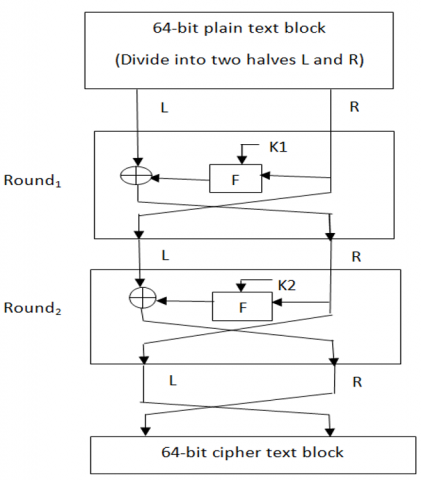Figure 4. Structure of proposed block cipher

## 5.png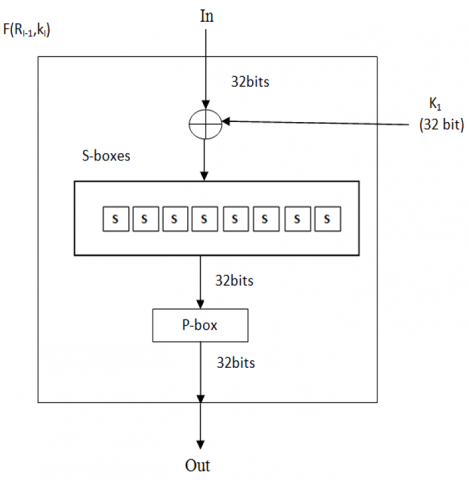Figure 5. F-function of proposed block cipher

The output bits from S-box are permuted with P-box  to get a new order of bits. Table 2 is P-box that shows the position where each bit moves. For example, 21 bit moves to bit 4, while bit 4 moves to bit 31.

Table 1. S-box

 x 0 1 2 3 4 5 6 7 8 9 A B C D E F S(x) C 5 6 B 9 0 A D 3 E F 8 4 7 1 2

Table 2. P-box
 bit 16 7 20 21 29 12 28 17 1 15 23 26 5 18 31 10 Goes to bit 1 2 3 4 5 6 7 8 9 10 11 12 13 14 15 16 bit 2 8 24 14 32 27 3 9 19 13 30 6 22 11 4 25 Goes to bit 17 18 19 20 21 22 23 24 25 26 27 28 29 30 31 32
Generation of hash value

Miyanguchi-praneel scheme is shown in Figure 6. H0 (initial checksum vector) is set to a fixed value and is used as a key for first iteration. It takes one chunk of message as plaintext (P) and encrypts it with a key (K). The ciphertext (C) obtained is XORED with the same message block and previous checksum to get new checksum. The new checksum will be the key for the next block and follow the same process until all blocks of message is processed. The output of the last block will be the final checksum. To make the algorithm stronger against attack, plaintext(P), cipher key and the cipher text are all exclusive-ored together to create the new digest.

The audio share is cleaved into N chunks each of 64 bits long. If last chunk is not 64 bits, it is padded with 1-bit followed by enough 0 bits to make it 64 bits. H0 (initial vector) is set to a fixed value and is used as a key for first iteration. The first block of the share goes through the block cipher as describe in Figure 4. Cipher text obtained will be XORed with same block and the key to produce a new 64 bit block. This block is the key for the next block of message and this process continues until last block of message is encrypted and XORed. The 64 bit from the last block is final checksum. The checksum generated is converted into decimal form and appended to the respective share. The audio share with appended checksum is then hidden into another image called shelter image by replacing the least significant bits of shelter image with the bits of the audio share. Stego-image is delivered to the participant. This conceals the existence of the share. This process is done for all n shares as shown in Figure 7.

## 6.pngFigure 6. Miyanguchi-praneel scheme

## 7.png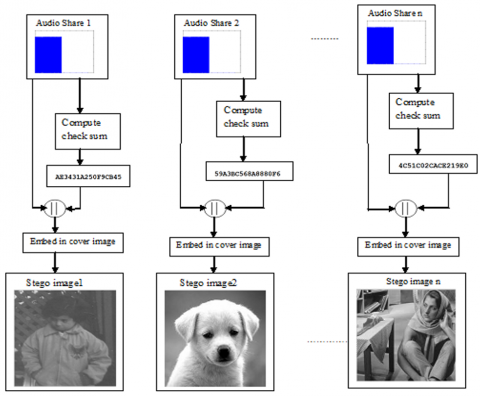Figure 7. Generation of checksum and embedding in cover image

Reconstruction of secret

Figure 8 shows extraction and verification of hash to determine its integrity. Before going for reconstruction of the secret, the participant has to first extract the share from the stego-image. Since the share is concatenated with checksum, share and the checksum are separated. Applying the same hash algorithm a checksum is created from the received share. Then check whether the calculated digest is same as the received hash value. If yes, no modifications have been done on the share and can go for reconstruction. The reconstruction process is shown in Figure 9. Otherwise the shares have been corrupted by some untrusted parties and so rejected.

## 8.png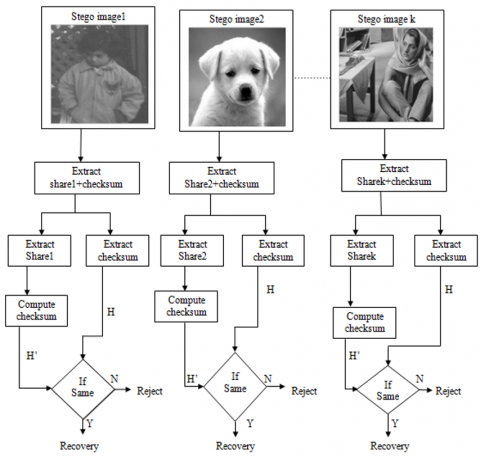Figure 8. Extraction and verification of checksum

## 9.pngFigure 9. Secret reconstruction

For secret reconstruction k or more than k participants has to submit their audio shares. Extract the first sampled values from k shares $s_{1}^{(1)}, s_{1}^{(2)}, s_{1}^{(3)}$, …. Use these values in equation given in step 2 of algorithm 6. Polynomial is derived on solving the equation and its coefficients excluding the leading coefficient are sampled values of secret audio. Same process is done for all sampled values of k audio shares to obtain the confidential audio.

Algorithm 6: Reconstruction of secret audio

Input: Secret shares S(1) , S(2), S(3), …, S(n)

Output: Secret audio A

1. Obtain any k audio shares that are to be used to reconstruct the audio A

2. Take the first sampled values $s_{j}^{(k)}$ from the k audio shares and j is the amplitude value and compute f(x)

\begin{aligned} f(x)=& s_{j}^{(1)} \frac{(x-2)(x-3) \ldots \ldots(x-k)}{(1-2)(1-3) \ldots \ldots(1-k)} \\ &+s_{j}^{(2)} \frac{(x-1)(x-3) \ldots \ldots(x-k)}{(2-1)(2-3) \ldots \ldots(2-k)}+\ldots \\ &+s_{j}^{(k)} \frac{(x-1)(x-2) \ldots \ldots(x-(k-1))}{(k-1)(k-2) \ldots .((k-(k-1))} \bmod 251 \end{aligned}

3. F(x) is rearranged and expressed as

4. The coefficients m0,m1,m2…mk-1 are the k-1 amplitude values  and store in A

5. Redo steps 2, 3 & 4 till all sampled values of the k audio shares are processed

6. Save A as secret audio

3. Results

The effect of proposed scheme is shown with an example of (2,3) threshold. Results achieved from the procedure explained in section 2.1, are laid out in Figure 10. Figure 10(a) is confidential audio and (b) is the encrypted audio. Figures 10(c) to (e) are shares generated and their size is half of the original audio. For reconstruction of any 2 shares are sufficient to get the secret. Figure 10(f) shows reconstructed secret after combining share1 and share 2. Figure 10(g) is the retrieved secret after decryption.

## 10.pngFigure 10. (a) Original audio; (b) encrypted audio; (c), (d) and (e) shares generated for participants 1, 2 & 3; (f) reconstructed audio after integrating share 1 and share 2; (g) decrypted audio

Results by implementing the same procedure to images are presented in Figure 11. Figure 11(a) & (b) are confidential image and its encrypted form. Figures 10(c) to (e) are shares generated with reduced dimensions equal to half of the original image. Figures 11(f) to (g) are the reconstructed and decrypted images.

## 11.pngFigure 11. (a) native image; (b) encrypted image; (c), (d) and (e) shares generated for participants 1, 2 & 3; (f) reconstructed image after combining (c) & (d); (g) decoded image

Results produced from the secret generation procedure describe in section 2.2 are displayed in Figure 12. Figure 12(a) is the original audio and 12(b) to (e) are shares generated with (3, 4) threshold scheme. For k=3, the shares generated are half size of original audio (1/k-1).

## 12.pngFigure 12. (3,4) threshold scheme; (a) Native audio; (b), (c), (d) and (e) shares generated for participants 1, 2, 3 & 4

## 13.png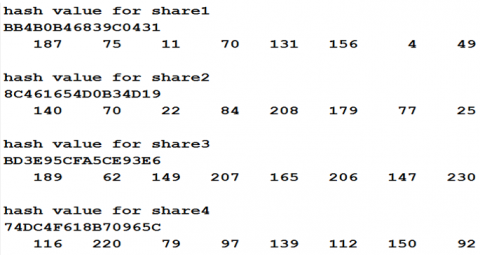Figure 13. Hash values for shares

## 14.pngFigure 14. (a) through (d) stego -images for participants 1, 2, 3 & 4

## 15.png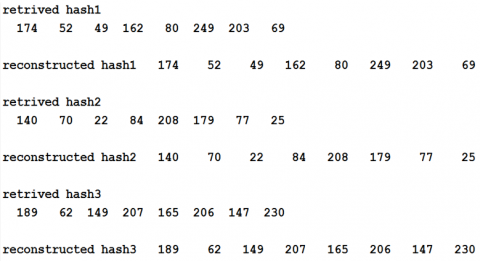Figure 15. Verification of checksum to determine integrity of shares

## 16.pngFigure 16. (a), (b) & (c) extracted shares from stego -images; (d) reconstructed audio

Hash function as described earlier is applied on the shares to generate checksum. Figure 13 shows checksum generated for shares in hexadecimal notation. These notations are converted into decimal form so as to concatenate with the shares. So it is evident that the proposed hash function make sure that a) hash algorithm can take input of different size and generates output of specified size b) knowing the hash value it is impossible to determine the shares (one way) c) for each share a different hash value is generated (collision resistant).

## 17.pngFigure 17. (3,4) threshold scheme; (a) original image; (b), (c), (d) and (e) shares generated for participants 1, 2, 3 & 4

## 18.pngFigure 18. Hash values for shares

## 19.png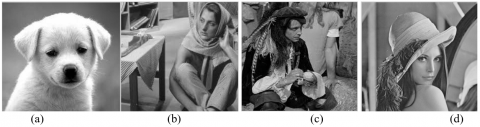Figure 19. (a) through (d) stego -images for participants 1, 2, 3 & 4

## 20.pngFigure 20. Verification of checksum to determine integrity of image shares

## 21.pngFigure 21. (a), (b) & (c) extracted shares from stego-images; (d) reconstructed image

After appending checksum to shares, each share is inserted into cover images using LSB Steganography and then sent to the participants. Figure 14 shows stego-images for participants 1 through 4.

Before restoring the secret, the participants must check the integrity of the shares. The extracted and recomputed checksum from the received shares are shown in Figure 15. Since both are same, they can go for reconstruction process.

The results in Figure 16 are for secret reconstruction described in algorithm 6. Figures (a) to (c) are 3 shares out of 4 that are to be combined to get the secret. Figure (d) shows the reconstructed audio after combining shares.

Original image and the shares generated with images for (3,4) threshold scheme are also shown in Figure 17. Dimensions of individual share are halved of original image.

Hash values generated for image shares are shown in Figure 18. Stego-images containing the shares are shown in Figure 19.

Figure 20 shows the retrieved hash values and the corresponding computed hash value and the reconstruction process is shown in Figure 21.

In terms of functionalities such as reduced share dimensions, integrity and Steganography our scheme is advantageous than some existing secret sharing schemes. In Shamir scheme  the share size is equal to size of secret where as our approach has reduced share size. Thenin and Lin  scheme generated shares of reduced size but the values of secret data greater than 250 are truncated into two values thereby increasing the size of secret data. Our scheme generates reduced share size without any truncation and also provides addition functionality of integrity and Steganography. In Wang et al. approach  shares are reduced to half size of secret whereas in our method reduction in size is based on threshold value. Higher threshold value smaller will be the share size.

4. Conclusion

In the proposed scheme, shares are generated in two different methods. In the first method, primarily original audio goes through encryption process and then shares are generated with the k amplitude values of the encrypted audio. Shares generated are reduced by 1/k dimensions of confidential audio. Likewise, burden of encryption and decryption can be reduced by having the xk-2 coefficients of the polynomial as the amplitude values and xk-1 coefficient as random number. In this case, dimensions of audio shares are 1/k-1 of confidential audio. Further the proposed scheme is equipped with a hash algorithm build on novel block cipher to protect integrity of shares. Experimental results for generation of hash value are shown for both audio and image and it is evident that none of the shares have same hash values and hence the hash algorithm is collision resistant. Finally, different cover images are taken and applying least significant bit replacement technique shares are concealed and then transmitted to the participants. Proposed scheme ensures that each individual share fails to disclose any details about the secret, each share size is reduced to either 1/k or 1/k-1 size of original audio or image and protect data from modifications by unauthorized parties.

References

 Shamir, A. (1979). How to share a secret. Communications of the ACM, 22(11): 612-613. https://doi.org/10.1145/359168.359176

 Blakley, G.R. (1979). Safeguarding cryptographic keys. In Proceedings of the AFIPS National Computer Conference, 1: 313-317. https://doi.org/10.1109/AFIPS.1979.98

 Naor, M., Shamir, A. (1995). Visual cryptography. In: De Santis A. (eds) Advances in Cryptology — EUROCRYPT'94. Lecture Notes in Computer Science, Springer, Berlin, Heidelberg, 950: 1-12. https://doi.org/10.1007/BFb0053419

 Ateniese, G., Blundo, C., De Santis, A., Stinson, D.R. (1996). Visual cryptography for general access structures. Information and Computation, 129(2): 86-106. https://doi.org/10.1006/inco.1996.0076

 Blundo, C., Santis, A.D., Stinson, D.R. (1999). On the contrast in visual cryptography schemes. Journal of Cryptology, 12(4): 261-289. https://doi.org/10.1007/s001459900057

 Hofmeister, T., Krause, M., Simon, H.U. (2000). Contrast-optimal k out of n secret sharing schemes in visual cryptography. Theoretical Computer Science, 240(2): 471-485. https://doi.org/10.1016/S0304-3975(99)00243-1

 Jin, D., Yan, W.Q., Kankanhalli, M.S. (2005). Progressive color visual cryptography. Journal of Electronic Imaging, 14(3): 033019. https://doi.org/10.1117/1.1993625

 Thien, C.C., Lin, J.C. (2002). Secret image sharing. Comput. Graphics, 26(5): 765-770. https://doi.org/10.1016/S0097-8493(02)00131-0

 Lin, C.C., Tsai, W.H. (2004). Secret image sharing with steganography and authentication. Journal of Systems and Software, 73(3): 405-414. https://doi.org/10.1016/S0164-1212(03)00239-5

 Yang, C.N., Chen, T.S., Yu, K.H., Wang, C.C. (2007). Improvements of image sharing with steganography and authentication. Journal of Systems and Software, 80(7): 1070-1076. https://doi.org/10.1016/j.jss.2006.11.022

 Lin, C.C., Tsai, W.H. (2003). Secret image sharing with capability of share data reduction. Optical Engineering, 42(8): 2340-2345. https://doi.org/10.1117/1.1588661

 Wang, R., Shyu, S. (2007). Scalable secret image sharing. Signal Processing: Image Communication, 22(4): 363-373. https://doi.org/10.1016/j.image.2006.12.012

 Yang, C., Huang, S. (2010). Constructions and properties of k out of n scalable secret image sharing. Optics Communications, 283(9): 1750-1762. https://doi.org/10.1016/j.optcom.2009.12.077

 Xie, D., Li, L.X., Peng, H.P., Yang, Y.X. (2017). A secure and efficient scalable secret image sharing scheme with flexible shadow sizes. PLOS ONE, Public Library of Science, 12(1): 1-17. https://doi.org/10.1371/journal.pone.0168674

 Yvo, D., Shuang, H., Jean-Jacques, Q. (1998). Audio and optical cryptography. Springer-Verlag Berlin Heidelberg, 1514: 392-404.

 Daniel, S., Spyros, S.M. (2005). General access structures in audio cryptography. IEEE International Conference on Electro Information Technology, Lincoln, NE, USA, 6. https://doi.org/10.1109/EIT.2005.1627018

 Li, H., Qin, Z., Zhang, X.P., Wang, X. (2011). Auditory cryptography security algorithm with audio shelters. Advanced in Control Engineering and Information Science- Procedia Engineering, 15: 2695-2699. https://doi.org/10.1016/j.proeng.2011.08.507

 Ehdaie, M., Eghlidos, T., Aref, M.R. (2008). A novel secret sharing scheme from audio perspective. 2008 International Symposium on Telecommunications, Tehran, Iran, pp. 13-18. https://doi.org/10.1109/ISTEL.2008.4651264

 Bogdanov, A., Knudsen, L.R., Leander, G., Paar, C., Poschmann, A., Robshaw, M.J.B., Seurin, Y., Vikkelsoe, C. (2007). PRESENT: An Ultra-Lightweight Block Cipher. Cryptographic Hardware and Embedded Systems, LNCS, Springer-Verlag Berlin Heidelberg, 450-466.

 Stalling, W. (2003). Cryptography and Network Security: Principles and Practices, Pearson Education Inc., 126-134.